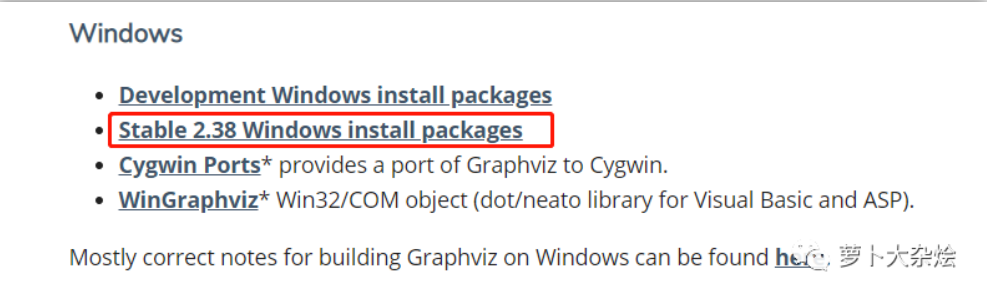# MATLAB 数据分析方法（第2版）1.4　数组和矩阵运算

+关注继续查看

1.4　数组和矩阵运算

1.4.1　数组的创建与运算

1.数组的创建

x=$a,b,c,d$

x=first：last

(续)

x=first：increment：last

x=linspace(first,last,n)

x=logspace(first,last,n)

x=$y,z,1,2,3$

y和z为数组，拼接y、z数组并扩展为更大的数组

2.数组元素的访问

3.标量与数组的运算

a+c=$a1+c,a2+c,…,an+c$即数组a的每个元素加上c

a*c或a.*c=$a1*c,a2*c,…,an*c$即数组a的每个元素乘以c

a/c或a./c=$a1/c,a2/c,…,an/c$即数组a的每个元素除以c

a.c=$c/a1,c/a2,…,c/an$即c除以数组a的每个元素

a.c=$a1c,a2c,…,anc$即数组a的每个元素的c次幂

c.a=$ca1,ca2,…,can$即以c为底，以a的每个元素为指数的幂

4.数组与数组的运算

a+b=$a1+b1,a2+b2,…,an+bn$即数组a与b的对应元素相加

a.*b=$a1*b1,a2*b2,…,an*bn$即数组a与b的对应元素相乘

a./b=$a1/b1,a2/b2,…,an/bn$即数组a与b的对应元素相除

a.b=$b1/a1,b2/a2,…,bn/an$即数组b与a的对应元素相除

a.b=$a1b1,a2b2,…,anbn$即数组a与b的对应元素的幂

dot(a,b)=a1*b1+a2*b2+…+an*bn即数组a与b的数量积或称向量内积

1.4.2　矩阵的输入与运算

1.矩阵的输入

1）直接输入法。从键盘直接输入矩阵的每一个元素。具体方法如下：将矩阵的所有元素用方括号括起来，在方括号内按矩阵行的顺序输入各元素，同一行的各元素之间用空格或逗号分隔，不同行的元素之间用分号或回车键分隔。例如：

>>A=$2,3,5;1,3,5;6,9,4$　　　　　　　%同一行元素之间用空格或逗号,行之间用分号或回车

A=

2　 3　 5

1　 3　 5

6　 9　 4

2）外部文件读入法。MATLAB语言允许用户调用在MATLAB环境之外定义的矩阵，可以利用任意文本编辑器所编辑的矩阵。矩阵元素之间以特定分断符分开，并按行列布置。load函数用于调用数据文件，其调用方法为：load+文件名$参数$。

1　　1　　 1

1　　2　　 3

1　　3　　 6

>> data1%显示数据

data1=

1　　1　　 1

1　　2　　 3

1　　3　　 6

（单位：元）

2016/3/18

3.43

3.44

3.4

3.42

0

2016/3/17

3.43

3.43

3.39

3.42

-0.2907

2016/3/16

3.44

3.44

3.37

3.37

1.7751

2016/3/15

3.38

3.4

3.34

3.4

-0.5882

2016/3/14

3.4

3.43

3.37

3.39

0

…

zgyh =

3.43　　3.44　　3.40　　3.42　 　 　　 0

3.43　　3.43　　3.39　　3.42　 -0.2907

3.44　　3.44　　3.37　　3.37 　　1.7751

3.38　　3.40　　3.34　　3.40 　-0.5882

3.40　　3.43　　3.37　　3.39　 　　　 0

3.40　　3.41　　3.33　　3.33　　1.4925

……

（余下数据省略了！）

2.特殊矩阵与符号矩阵

A=eye(m,n)

B=zeros(m,n)

C=ones(m,n)

D=magic(n)

E=vander(v)

F=hilb(n)

G=pascal(n)

H=rand(m)

randn(m)

syms x　　　　　　　　　　　　　　　%定义符号变量

f(x)=$x x^2;1+x^3 2+x^4$;%定义符号矩阵

a=f(3);%将x用3替换得数值矩阵a

3.矩阵中元素或块操作

A(k,：)

A(：,k)

A(：)

（续）

A(i1:i2,j1:j2)

A($a b c d$,:)

A(：,$e f g h$)

A(i2:-1：i1,：)

A(:,j2:-1：j1)

A(i1:i2,：)=

A(：,j1:j2)=

$A B$或$A；B$

diag(A,k)

tril(A,k)

triu(A,k)

flipud(A)

fliplr(A)

A’

rot90(A)

C=cat(DIM,A,B)

B=repmat(A,m,n)

K=kron(A,B)

B=reshape(A,m,n)

4.矩阵的运算

(1)矩阵间的运算

A+B(A-B)

A与B为同型矩阵，对应元素相加（减）

A*B

A的列数要等于B的行数，按代数学中定义的矩阵乘法法则计算

A/B

X=A/B是线性方程组XA=B的解。当A是可逆的矩阵时，A/B=A*B-1

AB

X=AB是线性方程组AX=B的解。当A是可逆的矩阵时，AB=A-1*B

A.*B

A与B为同型矩阵，对应元素相乘

A./B

A与B为同型矩阵，对应元素相除

A.B

A与B为同型矩阵，A中元素对应B中元素进行乘方运算

(2)矩阵与标量的运算

A+c(A-c)

A中每个元素加（减）常数c

A*c(c*A)

A中每个元素乘常数c

A/c

A中每个元素除常数c

（续）

c./A

c.A

A.c

Ac

A是方阵，当c大于0时表示矩阵的方幂，当c小于0时表示A逆的方幂

(3)矩阵的基本函数运算

det(A)

inv(A)

$m,n$=size(A)

$v,d$=eig(A)

A的特征向量v及特征值为对角元的对角矩阵d

orth(A)

rref(A)

rank(A)

trace(A)

$Q,R$=qr(A)

$p,H$=hess(A)

(4)矩阵的数据处理

MATLAB具有强大的数据处理功能，如数据的排序、求最大值、求和、求均值等。常用数据处理的命令见表1-17。

max(A)求向量或矩阵每列的最大值

mean(A)求向量或矩阵每列的平均值

sum(A)求向量或矩阵每列的元素和

var(A)求向量或矩阵每列的方差

cov(A)矩阵列向量之间的协方差矩阵

length(A)求向量所含元素个数

min(A)求向量或矩阵每列的最小值

median(A)求向量或矩阵每列的中位数

prod(A)求向量或矩阵每列的元素乘积

std(A)求向量或矩阵每列的标准差

corrcoef(A)矩阵列向量间的相关系数矩阵

find(A)求向量中满足条件的元素

5.多维数组

1）按“页”创建。

A(:,:,1)=$1,2,3;4,5,6;7,8,9$;　　　　　　　%创建数组A的第1页

A(:,:,2)=8;%创建数组A的第2页，每个元素赋值为8

2）由函数ones、zeros、rand直接创建。

>>A=rand(2,3,2)%创建A为2行、3列、2页的数组

A(:,:,1) =

0.8147　　0.1270　　0.6324

0.9058　　0.9134　　0.0975

A(:,:,2) =

0.2785　　0.9575　　0.1576

0.5469　　0.9649　　0.9706

3）由函数cat、repmat、reshape生成数组。

>>a=$1 2;3 4$;

>>b=$5 6;7 8$;

>>c=cat(3,a,b)%沿着第3维创建c为2行、2列、2页的数组

6.元胞数组

clear all;

a={$1 2 3;4 5 6;7 8 9$,20;ones(2,3),1:10}%创建元胞数组a

b=a{1,1}%从元胞数组中读取元素a{1,1}，该元素为矩阵赋给b

c=a{2,2}(3)%从元素a{2,2}读取第三个数据a{1,1}（3）

a{1,2}=magic(3)%给元素a{1,2}重新赋值为一个三阶魔方矩阵

a =

$3x3 double$　　$20$

$2x3 double$　　$1x10 double$

b =

1　　 2　　 3

4　　 5　　 6

7　　 8　　 9

c =

3

a =

$3x3 double$　　$3x3 double$

$2x3 double$　　$1x10 double$29 01105 0java后台创建url连接，获取接口数据

930 01429 0《Spark大数据分析实战》——3.4节MLlib
1566 0《图数据库（第2版）》导读
1513 01147 02014阿里巴巴校园招聘数据分析师职位笔试题目(答案版)
选择题10道，问答题三道，分析题2道 　 一、选择题 　　1、 一下哪个属于离散变量（汽车变量） 　　水稻亩产量 家庭收入 商品价格 汽车产量 　　2、 卡方分布的样本方差分别是（2n） 　    　n 1 2n 4n 　　3、 有个人买彩票，中奖概率为1/10,每次花200元，连续买5次，如果中奖则奖金为1000元，问不赔钱的概率是多少
3976 01469 0hash处理海量数据及其实现
【什么是Hash】   Hash，一般翻译做“散列”，也有直接音译为“哈希”的，就是把任意长度的输入（又叫做预映射， pre-image），通过散列算法，变换成固定长度的输出，该输出就是散列值。
882 0
10057

0

+ 订阅